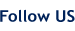# Fraction to Decimal Conversion Chart

 FRACTION TO DECIMAL CONVERSION CHART 1/64" = .0156 1/32" = .0313 3/64" = .0469 3/32" = .0938 5/64" = .0781 5/32" = .1563 7/64" = .1094 7/32" = .2188 9/64" = .1406 9/32" = .2813 11/64" = .1719 11/32" = .3438 13/64" = .2031 13/32" = .4063 15/64" = .2344 15/32" = .4688 17/64" = .2656 17/32" = .5313 19/64" = .2969 19/32" = .5938 21/64" = .3281 21/32" = .6563 23/64" = .3594 23/32" = .7188 25/64" = .3906 25/32" = .7813 27/64" = .4219 27/32" = .8438 29/64" = .4531 29/32" = .9.63 31/64" = .4844 31/32" = .9688 33/64" = .5156 35/64" = .5469 1/16" = .0625 37/64" = .5781 3/16" = .1875 39/64" = .6094 5/16" = .3125 41/64" = .6406 7/16" = .4375 43/64" = .6719 9/16" = .5625 45/64" = .7031 11/16" = .6875 47/64" = .7344 13/16" = .8125 49/64" = .7656 15/16" = .9375 51/64" = .7969 53/64" = .8281 1/8" = .125 55/64" = .8594 3/8" = .375 57/64" = .8906 5/8" = .625 59/64" = 9219 7/8" = .875 61/64" = .9531 1/4" = .25 63/64" = .9844 3/4" = .75 1/2" = .50

••••Friend Us